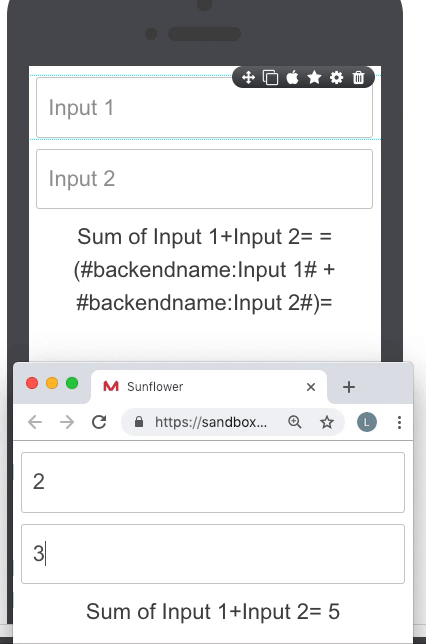##### Page tree
Go to start of banner

# Calculations & Math

Formulas are used to perform mathematical operations within app. It works at the FRONT of you app. Mobsted platform supports all arithmetical math functions and all MathJS formulas.

### Where Math is available

You can use Math formulas at ANY ELEMENT on the platform. For example - on Screens, in Elements Values or backend names, in Variables, on Elements hide/show conditions and in Actions, in Custom JS, basically anywhere where the platform need to work on any kind of text field.

The following notation activates math calculation on any of the elements - =(..... + .....)= , where simple math signs denote the operation needed. Numbers or data come into the same way, as with basic spreadsheets:

=( 5 + 100 )=   OR    =( 3 * 100 )=

For Functions wrap data source into additional parentecies, just like with Functions in spreadsheets, for example the square root funtion:

=( sqrt(4) )=

### Referencing data in Math

You can include Hashtags references to perform calculations with any data from any part of platform and build math expressions with it. For example:

=( #hashtag1# + #hashtag2# )=   OR    =( 3 + #hashtag2# )=

### Sample Use case

In the example below you can see that the Text field with the Formula has the =(........)= notation to sum of two data sources incoming from two inputs. ​So when user changes the input, the resulting calculation is also updated.### Examples of Available Math Formulas

The rest can be found here - https://mathjs.org/docs/index.html

 Action Example SUM / Multiply =( #hashtag1# + #hashtag2# )= / =( 5 * 2 )= Subtract / Divide =( #hashtag1# - #hashtag2# )= / =( 6 / 2 )= Modulus =( #hashtag1# % #hashtag2# )= Exponentiation =( #hashtag1# - #hashtag2# )= ^ =( #hashtag1# / #hashtag2# )= Square root =( sqrt(#hashtag1#) )= Factorial =( #hashtag1#! )=

### Live Demo of Math

You can also check the set up of some of the above functions on screen - 4 Math expressions & operations in Demo App in your profile.